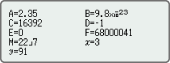# fx-82EX

Close

• The colors may differ slightly from the original.

## fx-82EX

Number of Functions : 274
Natural textbook display
Display expression same as textbook.
AAA × 1 (R03)

## Features

### Intuitive, easy-to-learn icon displayThe use of icons on the menu screen improves viewability. Selection of the desired function is quick and easy.

### Natural Textbook DisplayInput and display fractions, powers, logarithms, roots, and other mathematical formulas and symbols just as they appear in textbooks.

### List display function for thorough, speedy learningVariables and statistical calculation results stored in memory can be displayed in lists. There’s no need to recall and confirm individual values as with previous models.

### Easy-to-understand English messages and interactive formatNearly all English words are displayed on the screen in unabbreviated form. Interactive menu display realizes more intuitive operation.

### A comprehensive set of advanced functions unique to CASIO scientific calculators

#### Multiline displayFormulas are automatically wrapped to multiple lines. *
*In linear input mode

#### Variables listOne-touch list display

#### Statistics listDisplays six rows at once.

#### Digit separatorSeparation every three digits makes even large numbers easy to read.

## Specifications

• Non Programmable

•Number of Functions : 274

•Natural textbook display Display expression same as textbook.

•AAA × 1 (R03)

•10 + 2 digits 10-digit mantissa + 2-digit exponential display.

•Dot matrix display High-resolution screen provides beautiful looking graphs every time.

•Multi-replay Quick and easy recall of previously executed formulas for editing and re-execution.

•Plastic keys Designed and engineered for easy operation.

• Non Graphing

• AAA-size Battery

•List based STAT-data editor Viewing and editing of input data in list format, showing data groups (x-data, y-data, frequency) and surrounding data.

• Basic Statistics Standard statistics functions such as Mean, SUM, Standard Deviation, and Regression

• Basic Mathematical Functions Trigonometric, Exponential logarithmic, etc.

• Table Generation You can create a numerical table based on that expression by registering a function expression. In addition, graph functions can be represented by graphs.

• Intermediate Statistics More statistical functions such as Paired-variable statistical calculation, Quartiles and List display functions for speedy and thorough learning .